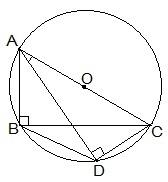Guru

# ABC and ADC are two right triangles with common hypotenuse AC. Prove that ∠ CAD = ∠CBD. Q.11

• 0

Give me the best way for solving the question of class 9th of Circles of exercise 10.5 of math. Give me the easiest way for solving this question in simple way of question no. 11 ABC and ADC are two right triangles with common hypotenuse AC. Prove that ∠ CAD = ∠CBD.

Share

1. We know that AC is the common hypotenuse and ∠ B = ∠ D = 90°.

Now, it has to be proven that ∠ CAD = ∠ CBDSince, ∠ ABC and ∠ ADC are 90°, it can be said that They lie in the semi-circle.

So, triangles ABC and ADC are in the semi-circle and the points A, B, C and D are concyclic.

Hence, CD is the chord of the circle with center O.

We know that the angles which are in the same segment of the circle are equal.

∴ ∠ CAD = ∠ CBD

• 0